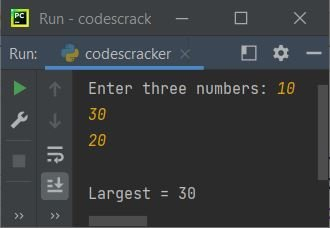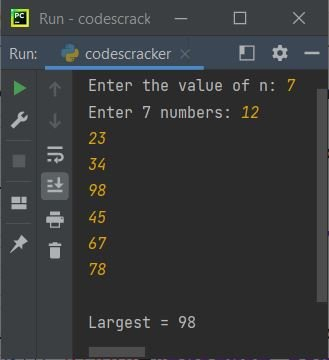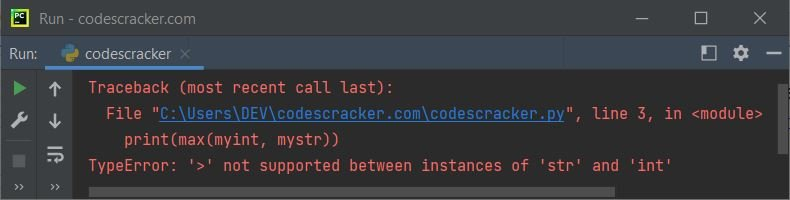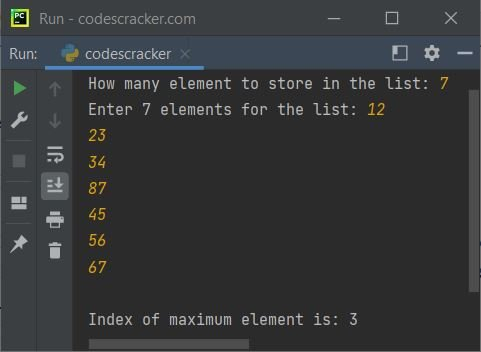# Python max() Function

The max() function in Python is used to find the largest item in an iterable, or the largest between/among two/multiple arguments. For example:

```mylist = [32, 34, 56, 76, 7]
print(max(mylist))

print(max(65, 76))
print(max(23, 45, 56, 67, 8, 100, 98))```

Output produced by above Python program, demonstrating the max() function, will exactly be:

```76
76
100```

## Python max() Function Syntax

Syntax to use max() function is:

`max(value1, value2, value3, ..., valueN, key)`

where value1, value2, and so on, upto valueN are arguments such as integers, floating-point numbers, strings etc.

While finding the maximum among multiple strings, the max() function returns the maximum string based on alphabetical-wise. Therefore, the key argument plays an important role in finding the maximum string based on length of all the string. Here is another syntax for iterable objects.

`max(iterable1, iterable2, ..., iterableN, key, default)`

Here iterable1, iterable2, and so on are iterable such as list, tuples, dictionary, set.

The default parameter is used to get the default as maximum value, if the iterable is empty.

Do not think much about above syntax. You'll get to know everything one by one, by example programs given below.

## Python max() Function Example

Let's create a simple example of max() function in Python:

```print("Enter three numbers: ", end="")
a = int(input())
b = int(input())
c = int(input())

print("\nLargest =", max(a, b, c))```

The snapshot given below shows the sample run of above program, with user input 10, 30, and 20 as three numbers:Now the problem is, what if user wants to enter n numbers to find and print the largest among all given n numbers ?
In that case we need a list to use. Here is the modified version of previous program:

```print("Enter the value of n: ", end="")
n = int(input())
print("Enter", n, "numbers: ", end="")
nums = []
for i in range(n):
num = int(input())
nums.append(num)

print("\nLargest =", max(nums))```

Here is its sample run with user input 12, 23, 34, 98, 45, 67, and 78 as seven numbers to find and print the largest among these seven numbers using the max() function:Important - If we pass multiple strings as arguments of max() to find the maximum string, then we get the maximum string based on alphabetical order, not by length. Let's take an example.

```maxStr = max("Anthony", "Alexander", "Alden", "David", "Daniel")
print(maxStr)```

Following is the output produced by this program:

`David`

Important - To find the largest string based on length, then use key argument with len as its value like shown in the example program given below.

```maxStr = max("Anthony", "Alexander", "Alden", "David", "Daniel", key=len)
print(maxStr)```

Now the output will be:

`Alexander`

because the word Alexander has highest number of characters among all the other 4 words.

The program given below is another example of max() function in Python:

```mylist = [12.4, 54.6, 1.43]
print(max(mylist))
print(max(12.32, 43, 54.65, 98.56, 32))
mystring = "codescracker"
print(max(mystring))
mydict = {"Name": "Ethan", "Region": "Maryland"}
print(max(mydict))```

Following is the output produced by this Python program:

```54.6
98.56
s
Region```

### How to Handle with Invalid Parameters of max() Function ?

The function max() raised an exception named TypeError when we pass arguments of multiple types. For example:

```myint = 12
mystr = "python"
print(max(myint, mystr))```

The output produced by this program is shown in the snapshot given below:So to handle these type of exceptions, we need to wrap the function in a try block, so that we can catch the raised exception using except block, as shown in the program given below:

```myint = 12
mystr = "python"
try:
print(max(myint, mystr))
except TypeError:
print("\nInvalid combination of arguments!")```

Now the output would be:

`Invalid combination of arguments!`

The above program can also be created in this way:

```myint = 12
mystr = "python"
try:
m = max(myint, mystr)
print(m)
except TypeError:
print("\nInvalid combination of arguments!")```

### Use max() to Find the Index of Maximum Element

The index of maximum element can also be calculated using the max() with index() function. Here is an example:

```print("How many element to store in the list: ", end="")
n = int(input())
print("Enter", n, "elements for the list: ", end="")
x = []
for i in range(n):
v = input()
x.append(v)

maxindex = x.index(max(x))
print("\nIndex of maximum element is:", maxindex)```

Sample run with user input 7 as size of list, 12, 23, 34, 87, 45, 56, 67 as seven elements is shown in the snapshot given below:## Python max() Function with default Argument

The default argument's value is considered as the maximum value, if the iterable is empty. Here is an example:

```mylist = []
print(max(mylist, default=50))```

The output produced by this program will exactly be:

`50`

## Python max() Function with Multiple Iterables

As shown in the syntax of max() function, we can also pass multiple iterables to find the iterable having maximum element. Here is an example demonstrates that version of the syntax:

```a = [12, 23, 34, 10]
b = [76, 8, 9, 10]
c = [1, 5, 7, 34]
print(max(a, b, c))```

The output produced by this program is given below:

`[76, 8, 9, 10]`

Because 76 is the highest number among all the numbers available in all the three lists. And the number 76 is available in the b list. Therefore the list b is printed on the output.

Python Online Test

« Previous Function Next Function »